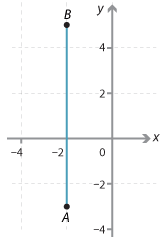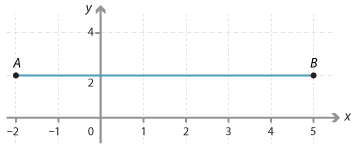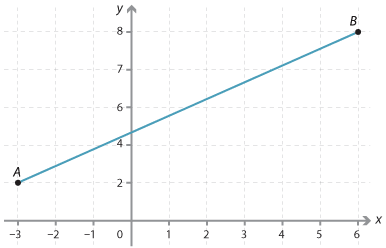#### Question 1

Find the midpoint of the following pairs of coordinates:

 a bA(−2, −3) and B(−2, 5) (−3, 1) (−2, 1) (−2, 0)A(−2, 2) and B(5, 2) (3, 2) (2, 3) (1$$\dfrac{1}{2}$$, 2)

#### Question 2

Find the coordinates of the midpoint of the line interval joining the following points:

 a bA(−3, 2) and B(6, 8) (7, $$\dfrac{1}{2}$$) (1$$\dfrac{1}{2}$$, 5) (4$$\dfrac{1}{2}$$, 2) A(−2, 8) and B(1, −1) (−$$\dfrac{1}{2}$$, 3$$\dfrac{1}{2}$$) (3, 0) (3$$\dfrac{1}{2}$$, $$\dfrac{1}{2}$$)

#### Question 3

The coordinates of the midpoint of the interval joining the points (−2, −5) and (3, 5) are:

 ($$-3\dfrac{1}{2}$$, 4) ($$\dfrac{1}{2}$$, 0) (2$$\dfrac{1}{2}$$, 5)